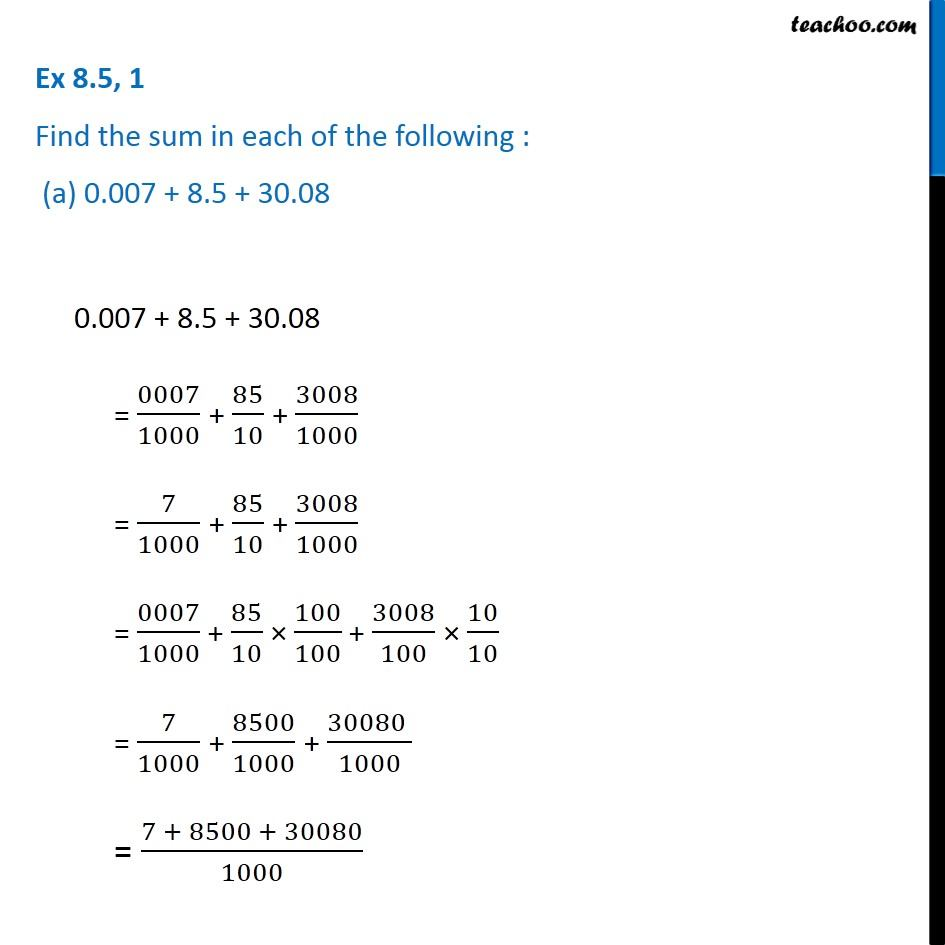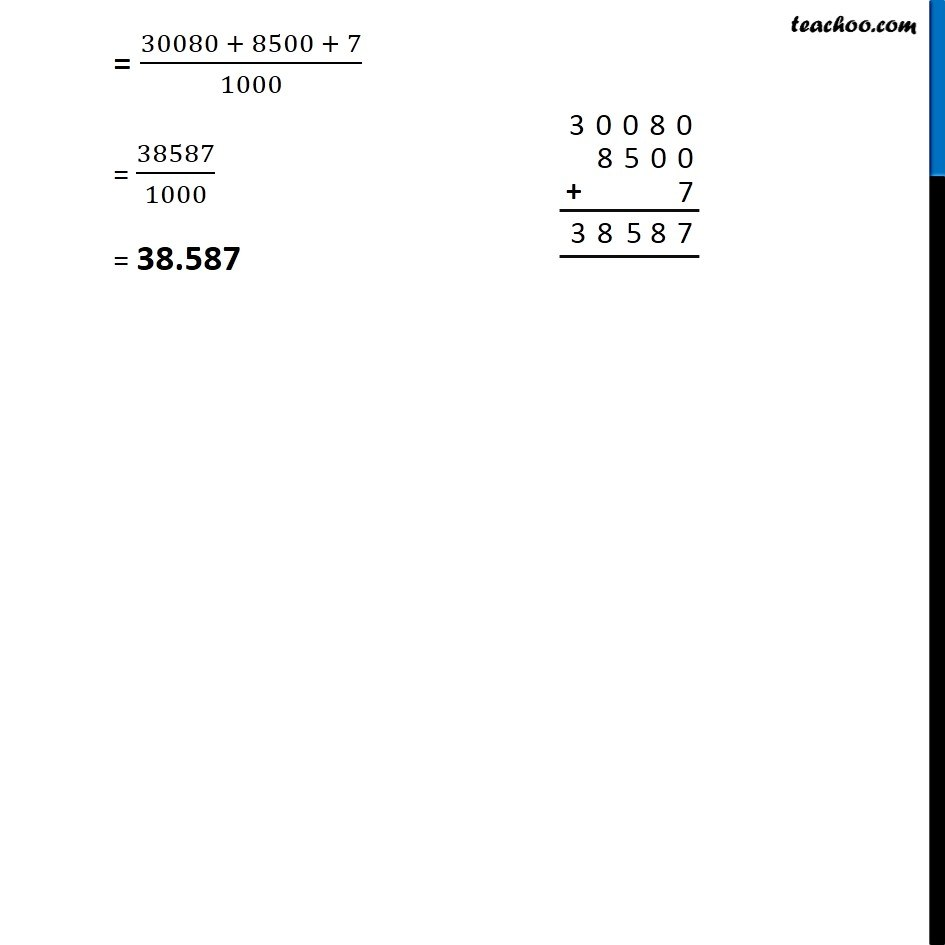1. Chapter 8 Class 6 Decimals
2. Concept wise

Transcript

Ex 8.5, 1 Find the sum in each of the following : (a) 0.007 + 8.5 + 30.080.007 + 8.5 + 30.08 = 0007/1000 + 85/10 + 3008/1000 = 7/1000 + 85/10 + 3008/1000 = 0007/1000 + 85/10 × 100/100 + 3008/100 × 10/10 = 7/1000 + 8500/1000 + (30080 )/1000 = (7 + 8500 + 30080)/1000 = (30080 + 8500 + 7)/1000 = 38587/1000 = 38.587